# PVOA Used in Recording a Transaction and Amortizing Discount

Let's illustrate how the calculation of the present value of an annuity is used in recording an accounting transaction.

### Exercise #11

On December 31, 2022, FreshStart provides a service for its customer DownCo in exchange for a promissory note requiring five annual payments of \$1,000 each. The payments are to occur on December 31 of each year beginning on December 31, 2023. The note does not specify any interest, and there is no market for the note. The value of the service performed by FreshStart is very unique and no market value is available. Based on the credit worthiness of DownCo and the length of the note, it is estimated that DownCo would have to pay 10% interest if it borrowed a similar amount from a bank. As FreshStart's accountant, how would you record the transaction on December 31, 2022?

Both the cost principle and the revenue recognition principle prohibit you from recording the \$5,000 as December 2022 revenue, since a portion of the \$5,000 is interest that will be earned in the five years 2023 through 2027. Since there is no fair market value (or cash equivalent amount) known for the note or for the service provided, you realize that the present value (or cash equivalent amount) of the note must be computed.

Your first step is to prepare a timeline that displays the relevant facts: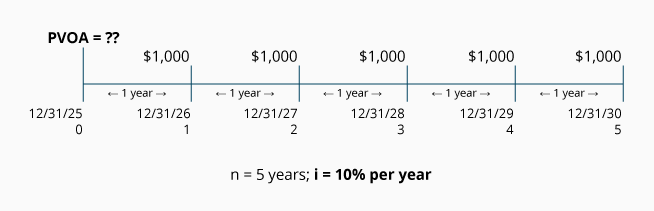Calculation of Exercise #11 using the PVOA Table

Using the information on the timeline, you insert the known figures into the PVOA equation: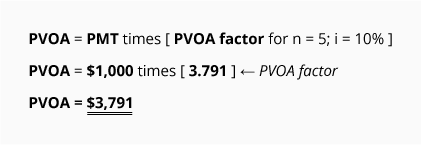This calculation tells you that receiving \$1,000 at the end of each of the next 5 years is the equivalent of \$3,791 at the present time if the time value of money is 10% per year. The difference between \$3,791 and the future amounts aggregating \$5,000 (5 payments at \$1,000 each) is \$1,209. This \$1,209 is interest that will be earned by FreshStart over the next five years. Expressed another way, FreshStart earned only \$3,791 in service revenues from DownCo on December 31, 2022.

A proof of this calculation is shown below:

Loan Amortization Schedule
(For \$3,791 at 10% per year with 5 annual payments.)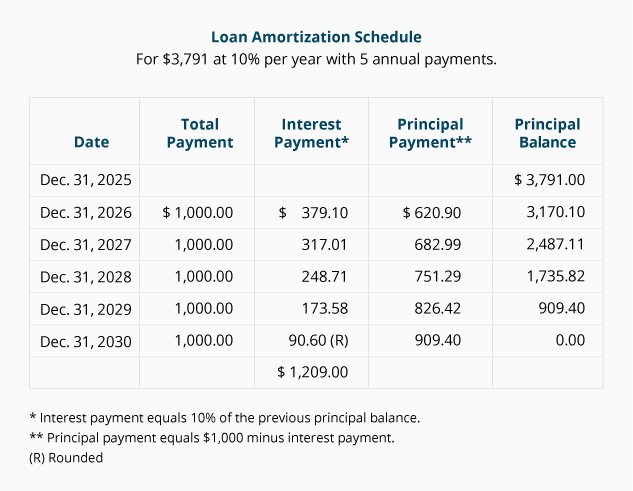FreshStart's journal entry to record this transaction on December 31, 2022 is: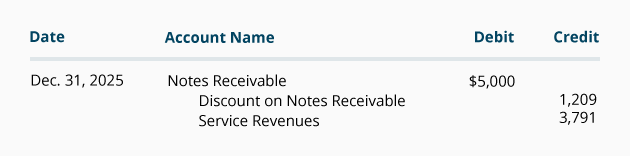The \$1,209 in Discount on Notes Receivable is to be amortized from this balance sheet account to the income statement account Interest Revenues over the life of the note.

If the discount and resulting interest revenue is immaterial in light of FreshStart's income statement, the discount can be amortized by using the straight-line method. Under the straight-line method, \$241.80 (\$1,209 divided by 5 years) will be recorded in each of the years 2023 through 2027, as shown in the following entry:If the discount and resulting interest revenue is material in light of FreshStart's income statement, generally accepted accounting principles requires that the discount be amortized by the effective interest rate method. The amounts for the effective interest rate method appear in the Interest Payment column of \$3,791 amortization schedule and are used in the following journal entries: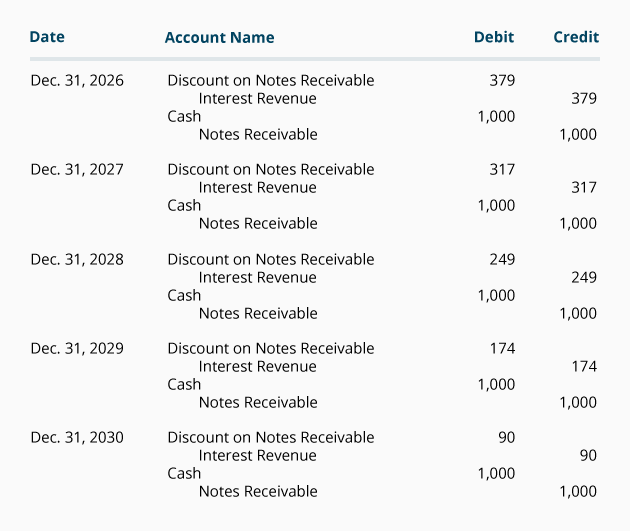After posting these journal entries, the book value (or carrying value) of this note receivable can be determined from the balances in the following T-accounts: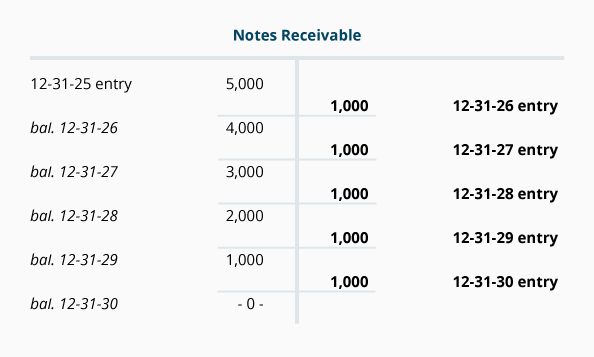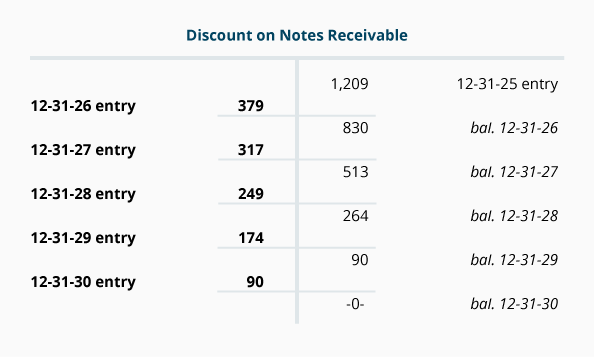The book value (or carrying value) of the notes receivable is calculated as shown here: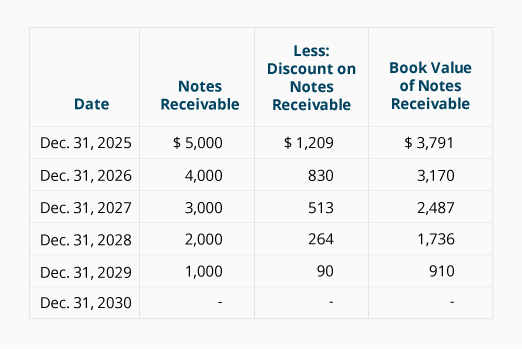Note that the book value amounts in the above exhibit are the same as the principal balance amounts in the Loan Amortization Schedule for \$3,791. If the notes receivable was written for \$3,791 with a stated interest of 10% and annual payments of \$1,000, the journal entries would have been: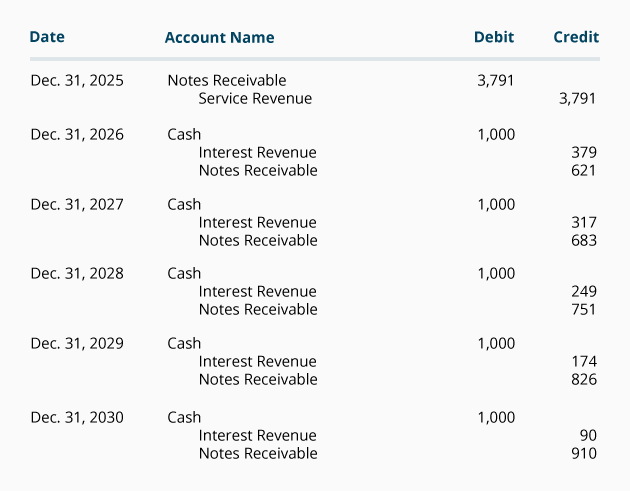#### Take Our Practice Quiz

We recommend that you now take our free Practice Quiz for this topic so that you can...

• See what you know
• See what you don't know